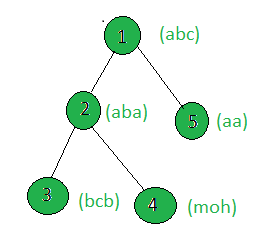Open in App
Not now

# Count the nodes of a tree whose weighted string does not contain any duplicate characters

• Difficulty Level : Medium
• Last Updated : 08 Jun, 2021

Given a tree, and the weights (in the form of strings) of all the nodes, the task is to count the nodes whose weights do not contain any duplicate character.
Examples:

Input:Output:
Only the strings of the node 1 and 4 contains unique strings.

Approach: Perform dfs on the tree and for every node, check if its string contains duplicate char or not, If not then increment the count.
Below is the implementation of the above approach:

## C++

 `// C++ implementation of the approach``#include ``using` `namespace` `std;` `int` `cnt = 0;` `vector<``int``> graph;``vector weight(100);` `// Function that returns true if the``// string contains unique characters``bool` `uniqueChars(string x)``{``    ``map<``char``, ``int``> mp;``    ``int` `n = x.size();` `    ``for` `(``int` `i = 0; i < n; i++)``        ``mp[x[i]]++;``    ``if` `(mp.size() == x.size())``        ``return` `true``;``    ``else``        ``return` `false``;``}` `// Function to perform dfs``void` `dfs(``int` `node, ``int` `parent)``{``    ``// If weighted string of the current``    ``// node contains unique characters``    ``if` `(uniqueChars(weight[node]))``        ``cnt += 1;` `    ``for` `(``int` `to : graph[node]) {``        ``if` `(to == parent)``            ``continue``;``        ``dfs(to, node);``    ``}``}` `// Driver code``int` `main()``{` `    ``// Weights of the nodes``    ``weight = ``"abc"``;``    ``weight = ``"aba"``;``    ``weight = ``"bcb"``;``    ``weight = ``"moh"``;``    ``weight = ``"aa"``;` `    ``// Edges of the tree``    ``graph.push_back(2);``    ``graph.push_back(3);``    ``graph.push_back(4);``    ``graph.push_back(5);` `    ``dfs(1, 1);` `    ``cout << cnt;` `    ``return` `0;``}`

## Java

 `// Java implementation of the approach``import` `java.util.*;` `class` `GFG``{` `    ``static` `int` `cnt = ``0``;` `    ``static` `Vector[] graph = ``new` `Vector[``100``];``    ``static` `String[] weight = ``new` `String[``100``];` `    ``// Function that returns true if the``    ``// String contains unique characters``    ``static` `boolean` `uniqueChars(``char``[] arr)``    ``{``        ``HashMap mp =``        ``new` `HashMap();``        ``int` `n = arr.length;` `        ``for` `(``int` `i = ``0``; i < n; i++)``            ``if` `(mp.containsKey(arr[i]))``            ``{``                ``mp.put(arr[i], mp.get(arr[i]) + ``1``);``            ``}``            ``else``            ``{``                ``mp.put(arr[i], ``1``);``            ``}``        ``if` `(mp.size() == arr.length)``            ``return` `true``;``        ``else``            ``return` `false``;``    ``}` `    ``// Function to perform dfs``    ``static` `void` `dfs(``int` `node, ``int` `parent)``    ``{``        ``// If weighted String of the current``        ``// node contains unique characters``        ``if` `(uniqueChars(weight[node].toCharArray()))``            ``cnt += ``1``;` `        ``for` `(``int` `to : graph[node])``        ``{``            ``if` `(to == parent)``                ``continue``;``            ``dfs(to, node);``        ``}``    ``}` `    ``// Driver code``    ``public` `static` `void` `main(String[] args)``    ``{` `        ``for` `(``int` `i = ``0``; i < ``100``; i++)``            ``graph[i] = ``new` `Vector();``        ` `        ``// Weights of the nodes``        ``weight[``1``] = ``"abc"``;``        ``weight[``2``] = ``"aba"``;``        ``weight[``3``] = ``"bcb"``;``        ``weight[``4``] = ``"moh"``;``        ``weight[``5``] = ``"aa"``;` `        ``// Edges of the tree``        ``graph[``1``].add(``2``);``        ``graph[``2``].add(``3``);``        ``graph[``2``].add(``4``);``        ``graph[``1``].add(``5``);` `        ``dfs(``1``, ``1``);` `        ``System.out.print(cnt);``    ``}``}` `// This code is contributed by Rajput-Ji`

## Python3

 `# Python3 implementation of the approach``cnt ``=` `0` `graph ``=` `[[] ``for` `i ``in` `range``(``100``)]``weight ``=` `[``0``] ``*` `100` `# Function that returns true if the``# string contains unique characters``def` `uniqueChars(x):``    ``mp ``=` `{}``    ``n ``=` `len``(x)``    ``for` `i ``in` `range``(n):``        ``if` `x[i] ``not` `in` `mp:``            ``mp[x[i]] ``=` `0``        ``mp[x[i]] ``+``=` `1``    ``if` `(``len``(mp) ``=``=` `len``(x)):``        ``return` `True``    ``else``:``        ``return` `False` `# Function to perform dfs``def` `dfs(node, parent):``    ``global` `cnt, x``    ` `    ``# If weight of the current node``    ``# node contains unique characters``    ``if` `(uniqueChars(weight[node])):``        ``cnt ``+``=` `1``    ``for` `to ``in` `graph[node]:``        ``if` `(to ``=``=` `parent):``            ``continue``        ``dfs(to, node)` `# Driver code``x ``=` `5` `# Weights of the node``weight[``1``] ``=` `"abc"``weight[``2``] ``=` `"aba"``weight[``3``] ``=` `"bcb"``weight[``4``] ``=` `"moh"``weight[``5``] ``=` `"aa"` `# Edges of the tree``graph[``1``].append(``2``)``graph[``2``].append(``3``)``graph[``2``].append(``4``)``graph[``1``].append(``5``)` `dfs(``1``, ``1``)``print``(cnt)` `# This code is contributed by SHUBHAMSINGH10`

## C#

 `// C# implementation of the approach``using` `System;``using` `System.Collections.Generic;` `class` `GFG``{` `    ``static` `int` `cnt = 0;` `    ``static` `List<``int``>[] graph = ``new` `List<``int``>;``    ``static` `String[] weight = ``new` `String;` `    ``// Function that returns true if the``    ``// String contains unique characters``    ``static` `bool` `uniqueChars(``char``[] arr)``    ``{``        ``Dictionary<``char``, ``int``> mp =``        ``new` `Dictionary<``char``, ``int``>();``        ``int` `n = arr.Length;` `        ``for` `(``int` `i = 0; i < n; i++)``            ``if` `(mp.ContainsKey(arr[i]))``            ``{``                ``mp[arr[i]] = mp[arr[i]] + 1;``            ``}``            ``else``            ``{``                ``mp.Add(arr[i], 1);``            ``}``        ``if` `(mp.Count == arr.Length)``            ``return` `true``;``        ``else``            ``return` `false``;``    ``}` `    ``// Function to perform dfs``    ``static` `void` `dfs(``int` `node, ``int` `parent)``    ``{``        ``// If weighted String of the current``        ``// node contains unique characters``        ``if` `(uniqueChars(weight[node].ToCharArray()))``            ``cnt += 1;` `        ``foreach` `(``int` `to ``in` `graph[node])``        ``{``            ``if` `(to == parent)``                ``continue``;``            ``dfs(to, node);``        ``}``    ``}` `    ``// Driver code``    ``public` `static` `void` `Main(String[] args)``    ``{` `        ``for` `(``int` `i = 0; i < 100; i++)``            ``graph[i] = ``new` `List<``int``>();``        ` `        ``// Weights of the nodes``        ``weight = ``"abc"``;``        ``weight = ``"aba"``;``        ``weight = ``"bcb"``;``        ``weight = ``"moh"``;``        ``weight = ``"aa"``;` `        ``// Edges of the tree``        ``graph.Add(2);``        ``graph.Add(3);``        ``graph.Add(4);``        ``graph.Add(5);` `        ``dfs(1, 1);` `        ``Console.Write(cnt);``    ``}``}` `// This code is contributed by PrinciRaj1992`

## Javascript

 ``

Output:

`2`

Complexity Analysis:

• Time Complexity: O(N*Len) where Len is the maximum length of the weighted string of a node in the given tree.
In dfs, every node of the tree is processed once and hence the complexity due to the dfs is O(N) if there are total N nodes in the tree. Also, processing of every node involves traversing the weighted string of that node thus adding a complexity of O(Len) where Len is the length of the weighted string. Therefore, the time complexity is O(N*Len).
• Auxiliary Space: O(1).
Any extra space is not required, so the space complexity is constant.

My Personal Notes arrow_drop_up